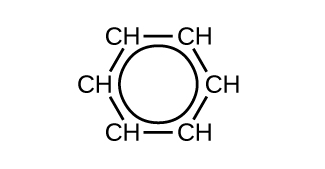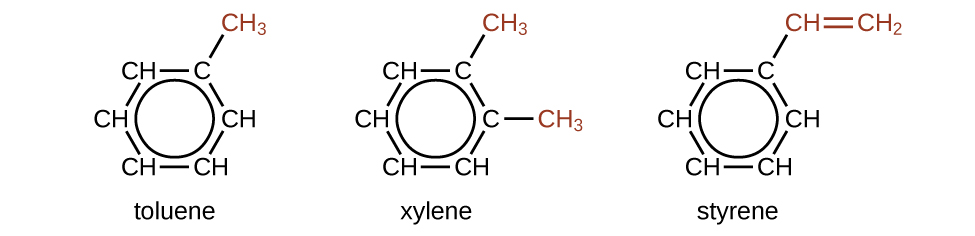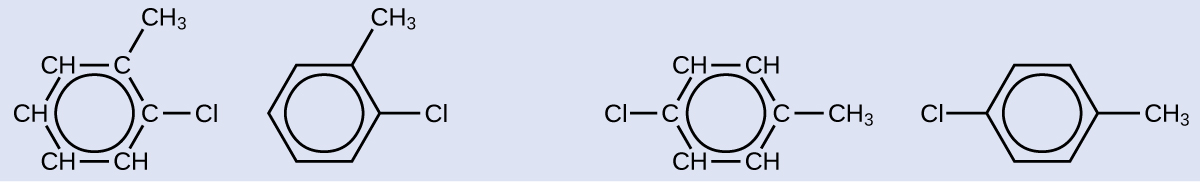# 22.7: Aromatic Hydrocarbons

$$\newcommand{\vecs}{\overset { \rightharpoonup} {\mathbf{#1}} }$$ $$\newcommand{\vecd}{\overset{-\!-\!\rightharpoonup}{\vphantom{a}\smash {#1}}}$$$$\newcommand{\id}{\mathrm{id}}$$ $$\newcommand{\Span}{\mathrm{span}}$$ $$\newcommand{\kernel}{\mathrm{null}\,}$$ $$\newcommand{\range}{\mathrm{range}\,}$$ $$\newcommand{\RealPart}{\mathrm{Re}}$$ $$\newcommand{\ImaginaryPart}{\mathrm{Im}}$$ $$\newcommand{\Argument}{\mathrm{Arg}}$$ $$\newcommand{\norm}{\| #1 \|}$$ $$\newcommand{\inner}{\langle #1, #2 \rangle}$$ $$\newcommand{\Span}{\mathrm{span}}$$ $$\newcommand{\id}{\mathrm{id}}$$ $$\newcommand{\Span}{\mathrm{span}}$$ $$\newcommand{\kernel}{\mathrm{null}\,}$$ $$\newcommand{\range}{\mathrm{range}\,}$$ $$\newcommand{\RealPart}{\mathrm{Re}}$$ $$\newcommand{\ImaginaryPart}{\mathrm{Im}}$$ $$\newcommand{\Argument}{\mathrm{Arg}}$$ $$\newcommand{\norm}{\| #1 \|}$$ $$\newcommand{\inner}{\langle #1, #2 \rangle}$$ $$\newcommand{\Span}{\mathrm{span}}$$$$\newcommand{\AA}{\unicode[.8,0]{x212B}}$$

Textbook, Hydrocarbons

Textbook, Hydrocarbons

Benzene, C6H6, is the simplest member of a large family of hydrocarbons, called aromatic hydrocarbons. These compounds contain ring structures and exhibit bonding that must be described using the resonance hybrid concept of valence bond theory or the delocalization concept of molecular orbital theory. (To review these concepts, refer to the earlier chapters on chemical bonding). The resonance structures for benzene, C6H6, are:Benzene is shown as a hexagonal ring. There are three double bonds in total. The left structure shows double bonds occupying three sides of the hexagon. A double sided arrow points to the right structure which has three double bonds which occupy the three previously unoccupied sides on the left.

Valence bond theory describes the benzene molecule and other planar aromatic hydrocarbon molecules as hexagonal rings of sp2-hybridized carbon atoms with the unhybridized p orbital of each carbon atom perpendicular to the plane of the ring. Three valence electrons in the sp2 hybrid orbitals of each carbon atom and the valence electron of each hydrogen atom form the framework of σ bonds in the benzene molecule. The fourth valence electron of each carbon atom is shared with an adjacent carbon atom in their unhybridized p orbitals to yield the π bonds. Benzene does not, however, exhibit the characteristics typical of an alkene. Each of the six bonds between its carbon atoms is equivalent and exhibits properties that are intermediate between those of a C–C single bond and a $$\mathrm{C=C}$$ double bond. To represent this unique bonding, structural formulas for benzene and its derivatives are typically drawn with single bonds between the carbon atoms and a circle within the ring as shown in Figure $$\PageIndex{10}$$.Figure $$\PageIndex{10}$$: This condensed formula shows the unique bonding structure of benzene.

There are many derivatives of benzene. The hydrogen atoms can be replaced by many different substituents. Aromatic compounds more readily undergo substitution reactions than addition reactions; replacement of one of the hydrogen atoms with another substituent will leave the delocalized double bonds intact. The following are typical examples of substituted benzene derivatives:Toluene has one of the H replaced by C H subscript 3 molecule. Xylene has 2 of the H atoms replaced by two C H subscript 3 molecules respectively. Styrene has one of the H atom replaced C H double bond C H subscript 2.

Toluene and xylene are important solvents and raw materials in the chemical industry. Styrene is used to produce the polymer polystyrene.

##### Structure of Aromatic Hydrocarbons

One possible isomer created by a substitution reaction that replaces a hydrogen atom attached to the aromatic ring of toluene with a chlorine atom is shown here. Draw two other possible isomers in which the chlorine atom replaces a different hydrogen atom attached to the aromatic ring:A hexagonal structure of benzene is shown with one of the H replaced by a chlorine atom and another H replaced by C H subscript 3. These two substitutions are apart by 1 carbon atom. Two structures are shown, one shows all the C and H in the ring written out, while the other omits it.
###### Solution

Since the six-carbon ring with alternating double bonds is necessary for the molecule to be classified as aromatic, appropriate isomers can be produced only by changing the positions of the chloro-substituent relative to the methyl-substituent:A hexagonal structure of benzene is shown with one of the H replaced by a chlorine atom and another H replaced by C H subscript 3. There are two of these isomers. The first isomer has these two substitutions adjacent to one another while the other are apart by 2 carbons. Two structures are shown for each isomer, one shows all the C and H in the ring written out, while the other omits it.
##### Exercise $$\PageIndex{7}$$

Draw three isomers of a six-membered aromatic ring compound substituted with two bromines.